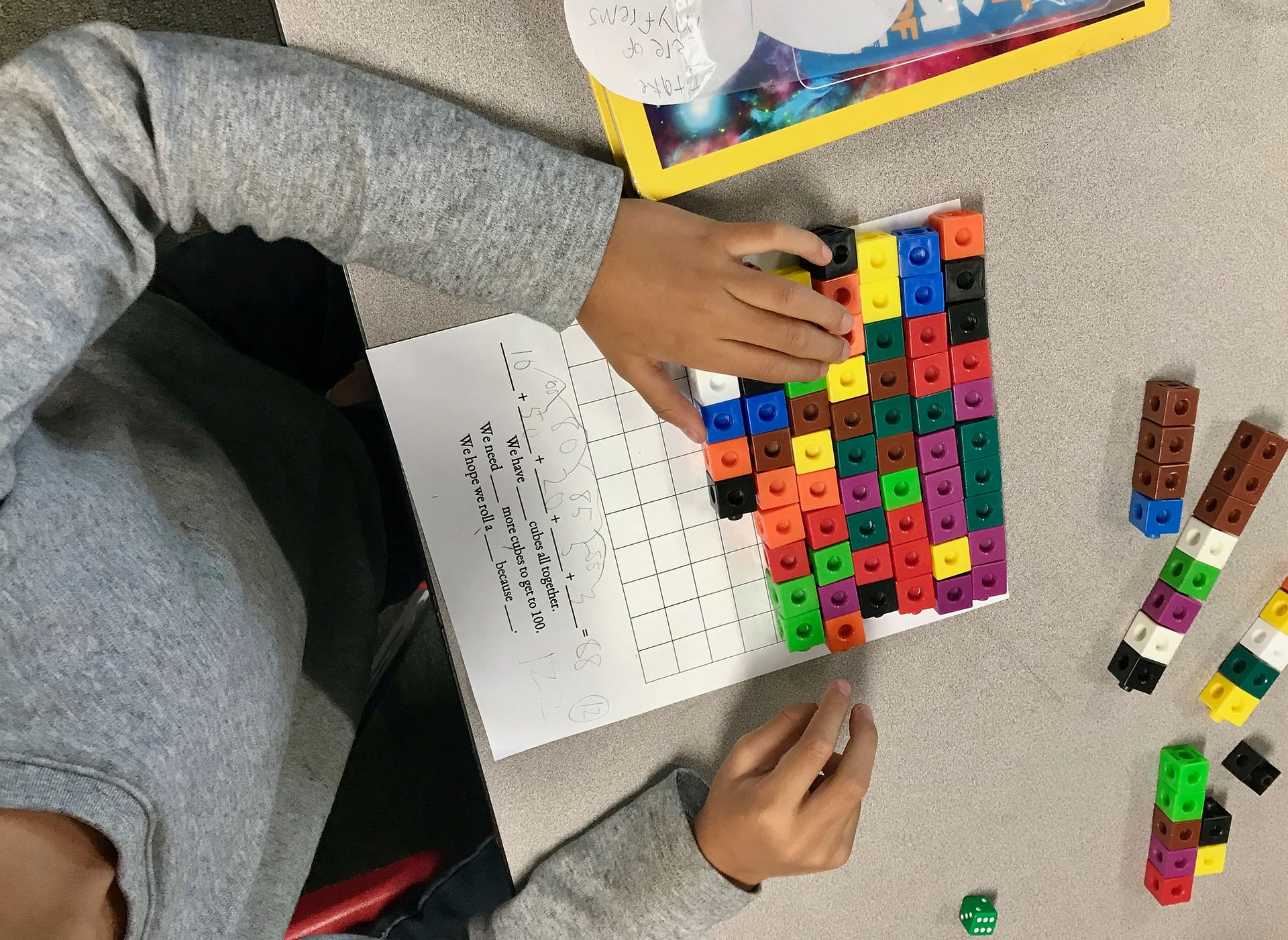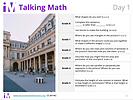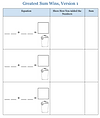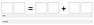### Math Warm-Ups(From Steve Wyborney)

Splat through 10:

Splat 1.1

Splat 1.2

Splat 1.3

Splat 1.4

Splat 1.5

Splat through 20

Splat 2.1

Multiple Splats

Splat 3.1Talking Math

(from Illustrative Math)

Visuals to use for synchronous class discussions.# Guess My Number Routine# Math Problem of the Day

Number and Operations in Base Ten: Addition and Subtraction

Common Core StandardsTen Frame Game

The four games that can be played with this applet help to develop counting and addition skills.

Students select a target number between 10-20 and find missing addends to make the target number.

Deep Sea DuelStudents use their knowledge of addition, place value, and strategic thinking to try to get as close to 1,000 as possible.

Close to 1,000 Video Demo

Digit Place

Lugar del Dígito (Spanish)

Students use their knowledge of addition, place value, and deductive reasoning to determine what the secret two-digit number is.

Digit Place Video Demo

Get to Zero

Llegue a Cero (Spanish)

Students practice subtraction within 1,000 as they race to get to zero.

Greatest Difference Wins

La Diferencia Más Grande Gana (Spanish)

Students build procedural fluency with subtraction by finding the difference between 2-digit numbers or 3-digit numbers.

Greatest Sum Wins

La Suma Más Grande Gana (Spanish)

Students build procedural fluency with addition by finding the sum of  2-digit numbers or 3-digit numbers.

Open Middle Task: Create an Equation

Students use only the digits 1 to 7, at most one time each, to fill in the boxes to create a true equation.

Students use the digits 1 to 9 at most one time each, to fill in the boxes to make the smallest (or largest) sum.

Open Middle Task: Subtracting Two-Digit Numbers (Elementary)

Students use the digits 1 to 9 at most one time each, fill in the boxes to make the smallest (or largest) difference.

Students use the digits 0 to 9, at most one time each, to make a true statement.

The Whopper Jar

The Juggler